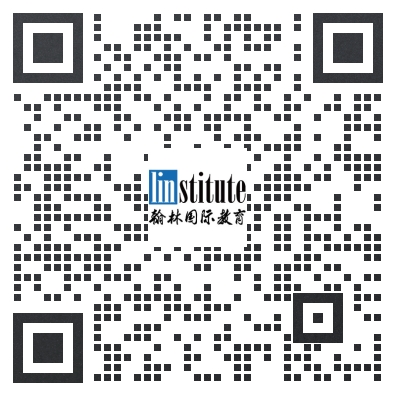# 2014 USAJMO Problems真题及答案

+答案解析请参考文末

## Day 1

### Problem 1

Let$a$$b$$c$ be real numbers greater than or equal to$1$. Prove that$$\min{\left (\frac{10a^2-5a+1}{b^2-5b+10},\frac{10b^2-5b+1}{c^2-5c+10},\frac{10c^2-5c+1}{a^2-5a+10}\right )}\leq abc.$$

### Problem 2

Let$\triangle{ABC}$ be a non-equilateral, acute triangle with$\angle A=60^\circ$, and let$O$ and$H$ denote the circumcenter and orthocenter of$\triangle{ABC}$, respectively.

(a) Prove that line$OH$ intersects both segments$AB$ and$AC$.

(b) Line$OH$ intersects segments$AB$ and$AC$ at$P$ and$Q$, respectively. Denote by$s$ and$t$ the respective areas of triangle$APQ$ and quadrilateral$BPQC$. Determine the range of possible values for$s/t$.

### Problem 3

Let$\mathbb{Z}$ be the set of integers. Find all functions$f : \mathbb{Z} \rightarrow \mathbb{Z}$ such that$$xf(2f(y)-x)+y^2f(2x-f(y))=\frac{f(x)^2}{x}+f(yf(y))$$for all$x, y \in \mathbb{Z}$ with$x \neq 0$.

## Day 2

### Problem 4

Let$b\geq 2$ be an integer, and let$s_b(n)$ denote the sum of the digits of$n$ when it is written in base$b$. Show that there are infinitely many positive integers that cannot be represented in the form$n+s_b(n)$, where$n$ is a positive integer.

### Problem 5

Let$k$ be a positive integer. Two players$A$ and$B$ play a game on an infinite grid of regular hexagons. Initially all the grid cells are empty. Then the players alternately take turns with$A$ moving first. In his move,$A$ may choose two adjacent hexagons in the grid which are empty and place a counter in both of them. In his move,$B$ may choose any counter on the board and remove it. If at any time there are$k$ consecutive grid cells in a line all of which contain a counter,$A$ wins. Find the minimum value of$k$ for which$A$ cannot win in a finite number of moves, or prove that no such minimum value exists.

### Problem 6

Let$ABC$ be a triangle with incenter$I$, incircle$\gamma$ and circumcircle$\Gamma$. Let$M,N,P$ be the midpoints of sides$\overline{BC}$$\overline{CA}$$\overline{AB}$ and let$E,F$ be the tangency points of$\gamma$ with$\overline{CA}$ and$\overline{AB}$, respectively. Let$U,V$ be the intersections of line$EF$ with line$MN$ and line$MP$, respectively, and let$X$ be the midpoint of arc$BAC$ of$\Gamma$.

(a) Prove that$I$ lies on ray$CV$.

(b) Prove that line$XI$ bisects$\overline{UV}$.

### 2014USAJMO真题参考答案及详解## 报名及辅导请联系